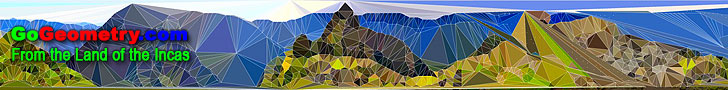# Welcome to GoGeometry: Recent additions (Page 13), Online LearningGo Geometry from the Land of the Incas - Recent Additions (Page 13 of 50)Geometry Problem 663. Scalene Triangle, 60 Degrees, Altitude, Angle Bisector, Perpendicular Bisector, Concurrent lines. Geometry Problem 662. Triangle, Incenter, Circumcenter, Orthocenter, Circle, Angle, Measurement. Geometry Problem 661. Intersecting Circles, Centers, Secant, Parallelogram. Geometry Problems 651 - 660. Archimedes Book of Lemmas, Circle, Triangle, Congruence, Parallelogram, Similarity. Geometry Problem 660. Triangle, Circle, Diameter, Perpendicular Lines. Geometry Problem 659. Parallelogram, Parallel Lines, Collinear Points. Geometry Problem 658. Triangle, Altitudes, Diameter, Circle, Angles. Geometry Problem 657. Triangle, Incircle, Tangency Points, Parallel Lines, Congruence. Geometry Problem 656. Triangle, Cevian, Concurrent Lines. Dynamic Geometry Software: GeoGebra 3.2 Help Document GeoGebra is a dynamic geometry software. Constructions can be made with points, vectors, segments, lines, polygons, conic sections, and functions. Interactive Mind Map Semicircle. Index. Theorems and Problems. Geometry Problem 655. Archimedes Book of Lemmas: Proposition 15 Circle, Inscribed Regular Pentagon, Radius. Geometry Problem 654. Archimedes Book of Lemmas: Proposition 14 Salinon, Semicircles, Diameter, Circle, Area. Geometry Problem 653. Archimedes Book of Lemmas: Proposition 13 Circle, Chord, Perpendicular, Congruence. Geometry Problems 641 - 650. Circle, Tangent, Semicircle, Perpendicular, Arbelos, Diameter. Geometry Problems 631 - 640. Triangle, Collinearity, Incenter, Circle, Arbelos. Go to Page: Previous | 1 | 2 | 3 | 4 | 5 | 6 | 7 | 8 | 9 | 10 | 11 | 12 | 13 | 14 | 15 | 16 | 17 | 18 | 19 | 20 | 21 | 22 | 23 | 24 | 25 | 26 | 27 | 28 | 29 | 30 | 31 | 32 | 33 | 34 | 35 | 36 | 37 | 38 | 39 | 40 | 41 | 42 | 43 | 44 | 45 | 46 | 47 | 48 | 49 | 50 | Next

 Home | GoGeometry Education | Geometry | Search | Email | By Antonio Gutierrez